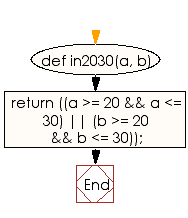﻿ Ruby Basic exercises: Check two integer values - w3resource# Ruby Basic Exercises: Check two integer values

## Ruby Basic: Exercise-8 with Solution

Write a Ruby program to check two integer values whether either of them is in the range 20..30 inclusive.

Ruby Code:

``````def in2030(a, b)
return ((a >= 20 && a <= 30) || (b >= 20 && b <= 30));
end

print in2030(15, 99),"\n"
print in2030(25, 28),"\n"
``````

Output:

```false
true
```

Flowchart:Ruby Code Editor: